# Analysis of Jan Panteltje's tritium decay data¶

### Mike Playle, 14th May 2020¶

For several years now Jan Panteltje has been logging the decay rate of a sample of tritium, in search of possible annual variations which have been hypothesised, but have never been demonstrated to exist (to the best of my knowledge).

His data is available to download from the link above, but very little analysis of it has been published. Here is my own meagre contribution. The results here should be reproducible given Jan's raw data files and an installation of Jupyter Notebook.

In the spirit of Jan's experiment, this analysis is released into the public domain.

First of all, we import the packages we will use.

In :
%matplotlib inline
import matplotlib.pyplot as plt
import numpy as np
from scipy import signal
from scipy.fftpack import fft
from math import *
import datetime

plt.rcParams['figure.figsize'] = [10, 5]


Now read and parse the raw data.

These data files are exactly as published on Jan's site.

I've assumed here that the experiment was restarted at exactly the same time of day every year, on the initial date shown in the file name. This might not be true; only Jan can clarify.

The earlier analysis by Paul McGill, also published on Jan's site, states that the first year of data was unreliable; my own attempts to analyse it seemed to confirm this, and so I've omitted it.

In :
files = ["tritium_decay_experiment_one_year_data_14_5_2013_to_14_5_2014.txt",
"tritium_decay_experiment_one_year_data_16_5_2014_to_16_5_2015.txt",
"tritium_decay_experiment_one_year_data_16_5_2015_to_16_5_2016.txt",
"tritium_decay_experiment_one_year_data_16_5_2016_to_16_5_2017.txt",
"tritium_decay_experiment_one_year_data_16_5_2017_to_14_5_2018.txt",
"tritium_decay_experiment_one_year_data_14_5_2018_to_14_5_2019.txt",
"tritium_decay_experiment_one_year_data_14_5_2019_to_13_5_2020.txt"]

time = []
light1 = []
light2 = []
reference = []
temperature = []

first_day = datetime.date(2013, 5, 14)

def parse_date(d):
dt = datetime.date(int(d[5:9]), int(d), int(d[:2]))
interval = dt - first_day
start_hours = interval.days * 24
print "Start date", dt, "hours:", start_hours
return start_hours

for name in files:
base = parse_date(name[39:48])
with open("Data/" + name, "r") as data:
for line in data:
line = line.strip().split(" ")
if len(line) == 12:
time.append(base + int(line))
light1.append(int(line))
reference.append(int(line))
light2.append(int(line))
temperature.append(int(line))


Start date 2013-05-14 hours: 0
Start date 2014-05-16 hours: 8808
Start date 2015-05-16 hours: 17568
Start date 2016-05-16 hours: 26352
Start date 2017-05-16 hours: 35112
Start date 2018-05-14 hours: 43824
Start date 2019-05-14 hours: 52584


The data measures time in hours, but we'll convert it to years for the subsequent analysis.

In :
time_years = [t / (365. * 24.) for t in time]


The reference and temperature data look flat at first, but they're actually "dithering" between adjacent integer values, so we can still hope to extract useful meaning from them by filtering (at the expense of time resolution, but we have plenty of that).

The Light1 and Light2 data look like exponential decay curves, as we should expect from the known behaviour of tritium, which is encouraging.

In :
plt.title("Raw tritium experiment data", fontsize=20)
plt.xlabel("Time (years)")
plt.plot(time_years, light1, label="Light1")
plt.plot(time_years, light2, label="Light2")
plt.plot(time_years, reference, label="Reference")
plt.plot(time_years, temperature, label="Temperature")
plt.legend()
plt.show()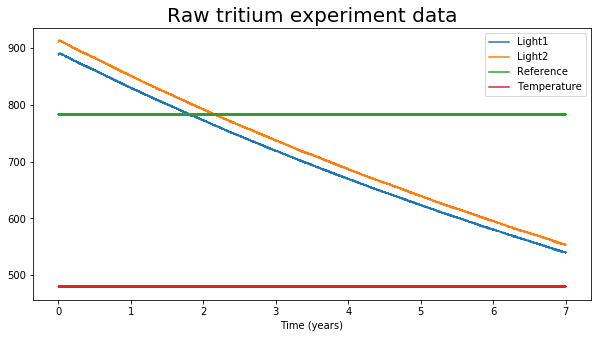The first 10 data points appear to be outliers, perhaps caused by the apparatus warming back up after being restarted, so I'll trim them off. Paul McGill observed this too. I didn't see any similar anomalies at the start of the other data files.

In :
start = 10
time = time[start:]
time_years = time_years[start:]
light1 = light1[start:]
light2 = light2[start:]
reference = reference[start:]
temperature = temperature[start:]


Next, fit an exponential decay to the Light1 and Light2 curves.

If

$$V_t = A_0e^{\frac{t}{\tau}}$$

then

$$\ln{V_t} = \ln{A_0} + \frac{1}{\tau} t$$

So this can be done by fitting a line to the logarithm of the data.

Once done, print the calculated half-lives and subtract the exponential from the data to leave the residuals.

In :
from scipy import stats

log_light1 = [log(v) for v in light1]
slope, intercept, r, p, err1 = stats.linregress(time_years, log_light1)
A0_1 = exp(intercept)
tau_1 = 1./slope

log_light2 = [log(v) for v in light2]
slope, intercept, r, p, err2 = stats.linregress(time_years, log_light2)
A0_2 = exp(intercept)
tau_2 = 1./slope

print "Light1: A0 =", A0_1, "half life =", tau_1 * log(0.5), "err:", err1
print "Light2: A0 =", A0_2, "half life =", tau_2 * log(0.5), "err:", err2

residual_1 = [val - A0_1*exp(t / tau_1) for (val, t) in zip(light1, time_years)]
residual_2 = [val - A0_2*exp(t / tau_2) for (val, t) in zip(light2, time_years)]

Light1: A0 = 892.097465388 half life = 9.664424648219581 err: 1.2039696546177393e-06
Light2: A0 = 914.583602002 half life = 9.673165614716432 err: 1.369976937137238e-06


It's interesting to see that the calculated values match each other, and Paul McGill's earlier numbers, fairly closely; however they are significantly smaller than the published half life of tritium (about 12.3 years).

Maybe the measured values aren't linear in the decay rate of the tritium? Nonlinearity in the phosphor, the photodetectors or the circuitry could cause this effect.

Anyway, let's look at the raw residuals. They're very noisy; some filtering will be called for later.

In :
plt.title("Tritium experiment - residuals", fontsize=20)
plt.plot(time_years, residual_1, label="Light1")
plt.plot(time_years, residual_2, label="Light2")
plt.legend()
plt.show()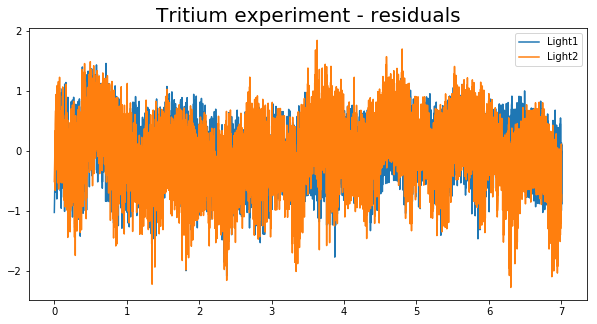Let's take a look in the frequency domain. We have 7 years of data so we can hope for a frequency resolution down to 1/7 per year. We only plot the lowest frequencies; the FFT results contain a lot of data for much higher frequencies, which are not of much interest to us here.

In :
N = len(residual_1)
window = signal.hamming(N)

# sample spacing
T = 1.0 / (365. * 24.)

spec1 = fft(residual_1 * window)
ampl1 = 2.0/N * np.abs(spec1[0:N//2])

spec2 = fft(residual_2 * window)
ampl2 = 2.0/N * np.abs(spec2[0:N//2])

s_ref = fft(reference * window)
a_ref = 2.0/N * np.abs(s_ref[0:N//2])

s_tmp = fft(temperature * window)
a_tmp = 2.0/N * np.abs(s_tmp[0:N//2])

xf = np.linspace(0.0, 1.0/(2.0*T), N//2)

nplot = 50
xf = xf[3:nplot]
ampl1 = ampl1[3:nplot]
ampl2 = ampl2[3:nplot]
a_ref = a_ref[3:nplot]
a_tmp = a_tmp[3:nplot]

plt.title("Tritium experiment - frequency domain", fontsize=20)

plt.xlabel("Frequency (per year)")
plt.plot(xf, ampl1, label="Light1")
plt.plot(xf, ampl2, label="Light2")
plt.plot(xf, a_ref * 3.0, label="Reference")
plt.plot(xf, a_tmp, label="Temperature")

plt.legend()
plt.show()

plt.title("Tritium experiment - frequency domain - temperature", fontsize=20)

plt.xlabel("Frequency (per year)")
plt.plot(xf, a_tmp, label="Temperature")
plt.legend()
plt.show()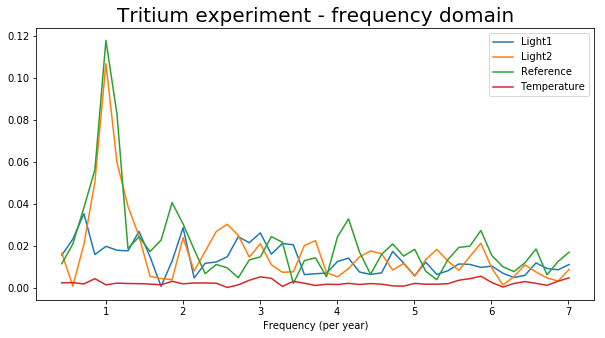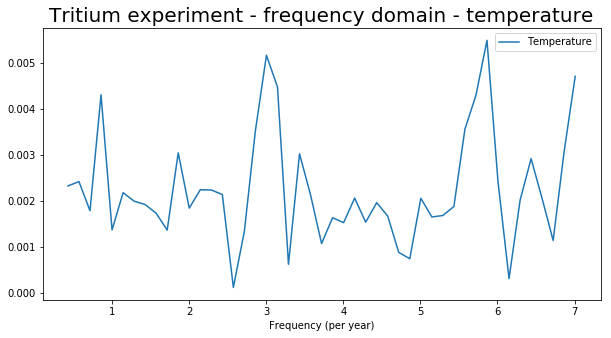There's a noticeable peak at 1/year in both the Light2 and reference values, but there's no significant yearly component to Light1. This suggests that the peak is due to something other than the behaviour of the tritium.

Let's look at the filtered residuals.

In :
def moving_average(x, w):
return np.convolve(x, np.ones(w), 'valid') / w

window = 1000
decimate = 200

r1_filtered = moving_average(residual_1, window)[::decimate]
r2_filtered = moving_average(residual_2, window)[::decimate]
temp_filtered = moving_average(temperature, window)[::decimate]
ref_filtered = moving_average(reference, window)[::decimate]
time_filtered = np.array(time_years)[::decimate][:len(r1_filtered)]

plt.title("Tritium experiment - filtered residuals", fontsize=20)
plt.plot(time_filtered, r1_filtered, label="Light1")
plt.plot(time_filtered, r2_filtered, label="Light2")
plt.legend()
plt.show()

plt.title("Tritium experiment - filtered residuals", fontsize=20)
plt.plot(time_filtered, temp_filtered, label="Temperature")
plt.legend()
plt.show()

plt.title("Tritium experiment - filtered residuals", fontsize=20)
plt.plot(time_filtered, ref_filtered, label="Reference")
plt.legend()
plt.show()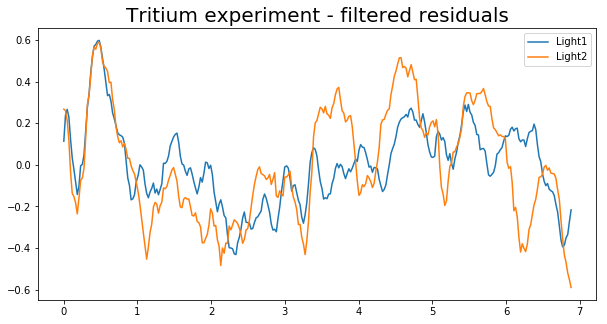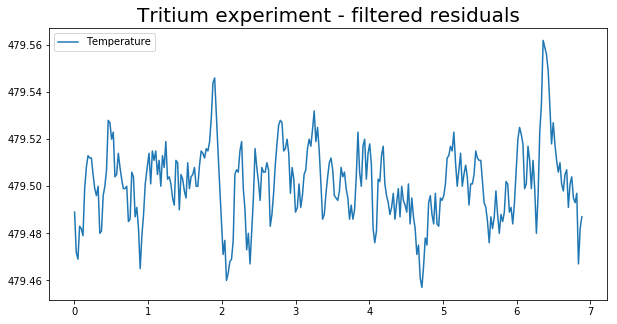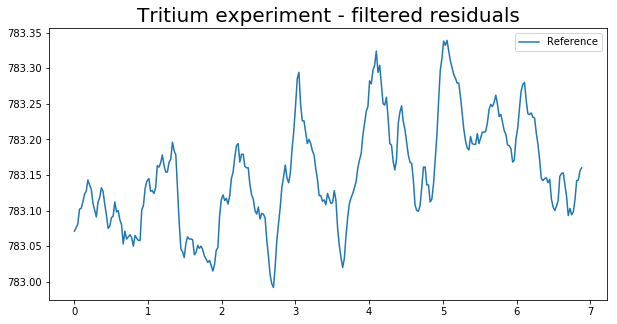Interestingly, during the first year the two light signals track each other closely, but later they diverge. This tends to suggest that we're seeing a property of the measurement apparatus rather than the tritium it contains. Did something change after t = 1 year?

Anyway, Jan's site suggests a possible correlation with the distance from the sun. This can be calculated using the Skyfield Python module.

There does appear to be a correlation with Light2 - but this is hardly surprising, given that the distance varies annually, and we've already identified an annual pattern in the data. Correlation is not causation.

In :
from skyfield.api import load

earth, sun = planets['earth'], planets['sun']

count = 0

def distance_to_sun(t):
global count
dt = datetime.date(2012, 11, 14) + datetime.timedelta(365.0*t)
position = earth.at(ts.utc(dt)).observe(sun)
count += 1
if count % 1000 == 0:
print count, "calculated"
return distance.au

sun_dists = [distance_to_sun(t) for t in time_filtered]
print len(sun_dists), "sun distances calculated"

302 sun distances calculated

In :
fig, ax1 = plt.subplots()
ax1.set_title("Tritium experiment - comparison with solar distance", fontsize=20)
ax1.set_xlabel("Time (years)")
ax1.set_ylabel("Distance to sun (AU)")
ax1.plot(time_filtered, sun_dists)

ax2 = ax1.twinx()
ax2.set_ylabel("Light residual")

ax2.plot(time_filtered, r1_filtered, color="red", label="Light1")
ax2.plot(time_filtered, r2_filtered, color="green", label="Light2")
plt.legend()
plt.show()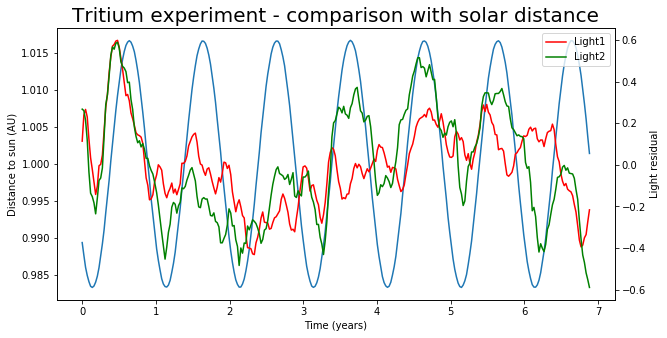In conclusion, while there does appear to be an annual component to these measurements, there are reasons to suspect that it arises from the behaviour of the measurement apparatus, and so this analysis neither confirms nor refutes the hypothesis that the decay rate of tritium varies annually.